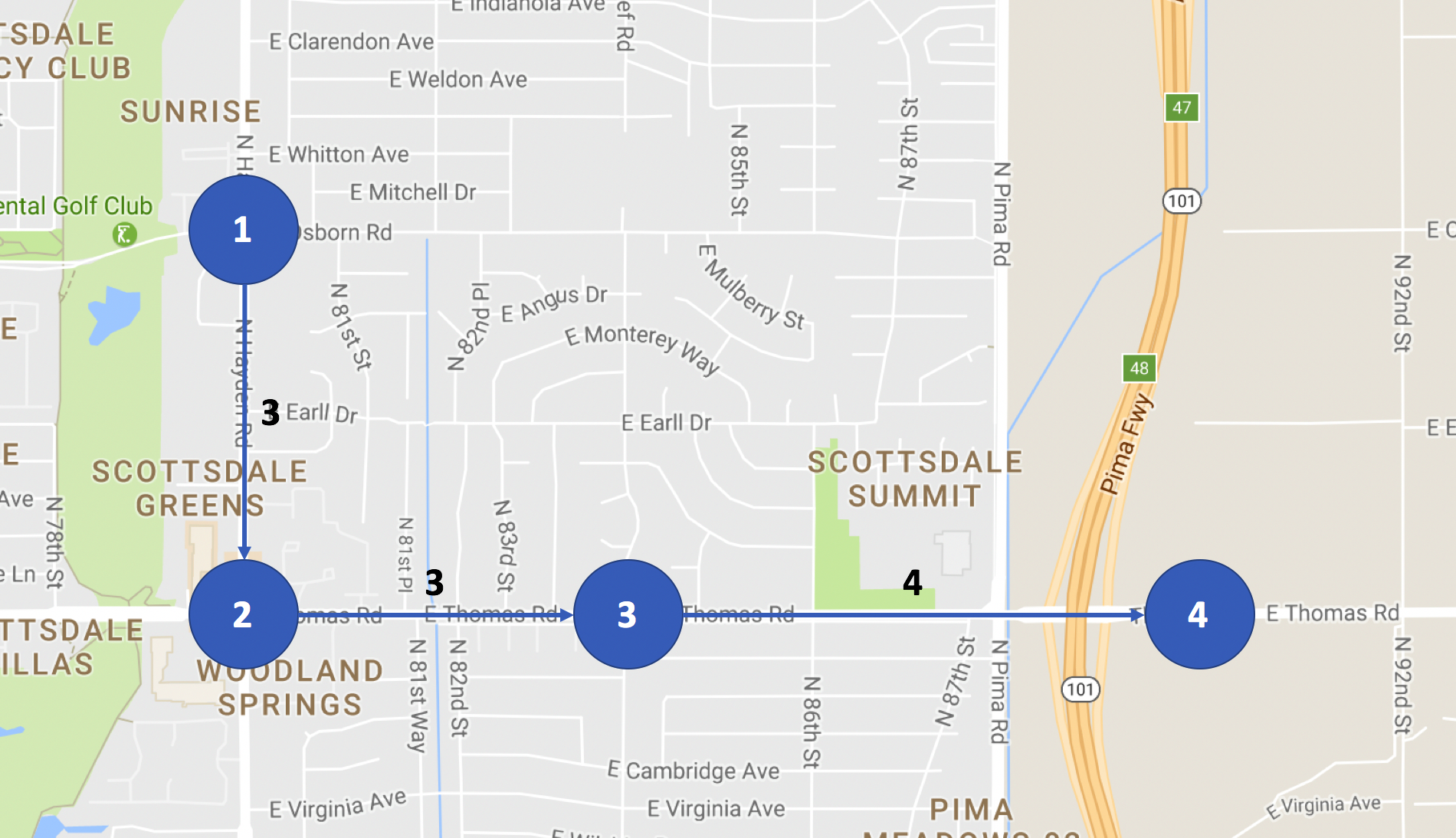Introduction to Graph Algorithm Implementations in Ruby

This guide walks through how to work with a mapping application and apply graph traversal techniques to find the distance for each weight in a graph.

By Jordan HudgensSummary

Build a method that finds the distance for each weight in a directed graph.

Code File

Exercise Description

Given a route on map, that contains stops that have a weight measured between each stop, and the total distance, figure out the value that each weight represents.

Example RouteExample Data

Given the total distance of:

15

And the three nodes, represented by an array:

[[1, 3], [2, 3], [3, 4]]

Expected Output

The value of each weight is:

1.5

Real World Usage

In this exercise, you'll learn how to work with graph analysis. In computer science, graphs give the ability to assign priorities and make connections between data points. Working with applications, such as mapping tools, are a common situation where working with graphs is required knowledge.

Solution

Can be found on the solutions branch on github.Jordan Hudgens

I've been a software engineer for the past decade and have traveled the world building applications and training individuals on a wide variety of topics.